###### Summary -

In this topic, we described about the Interest Calculation Types along with technical information.

Calculating interest on total balance of an account or total balance of customer account is called as interest calculation. Interest calculation could be either Item, Balance or Penalty type.

Interest calculation represented in "s". Interest calculation configuration can be done by two methods i.e Interest calculation on account balances and Interest calculation on areas.

## Steps for interest calculation are -

1 Define interest calculation type OB46 SPRO → SAP Reference IMG → Financial Accounting → General Ledger Accounting → Business Transactions → Bank Account Interest Calculation → Interest Calculation Global Settings → Define Interest Calculation Types
2 Prepare account balance interest calculation OBAA SPRO → SAP Reference IMG → Financial Accounting → General Ledger Accounting → Business Transactions → Bank Account Interest Calculation → Interest Calculation Global Settings → Prepare Account Balance Interest Calculation
3 Define reference interest rates OBAC SPRO → SAP Reference IMG → Financial Accounting → General Ledger Accounting → Business Transactions → Bank Account Interest Calculation → Interest Calculation → Define Reference Interest Rates
4 Define time dependent terms OB81 SPRO → SAP Reference IMG → Financial Accounting → General Ledger Accounting → Business Transactions → Bank Account Interest Calculation → Interest Calculation → Define Time Dependent Terms
5 Define/specify interest Values OB83 SPRO → SAP Reference IMG → Financial Accounting → General Ledger Accounting → Business Transactions → Bank Account Interest Calculation → Interest Calculation → Enter Interest Values
6 Creation of G/L Account FS00
7 Define automatic posting Account OBV2 SPRO → SAP Reference IMG → Financial Accounting → General Ledger Accounting → Business Transactions → Bank Account Interest Calculation → Interest Posting → Prepare G/L Account Balance Interest Calculation
8 Posting of term loan F-02
9 Repayment of term loan F-02
10 Interest Calculations F.52 Accounting → Financial Accounting → General Ledger → Periodic Processing → Interest Calculations → Balances

In this chapter, we will discuss about defining interest calculation types.

Step-1: Enter the transaction code OB46 in the SAP command field and click Enter to continue.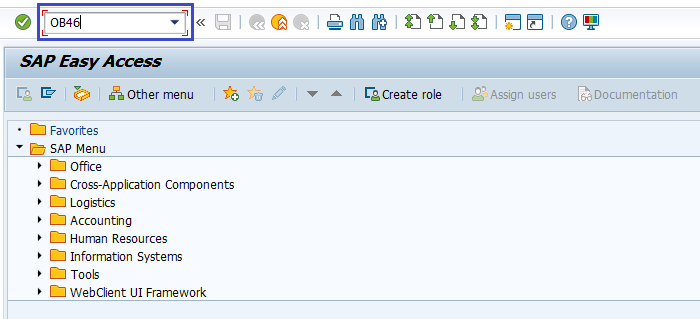(OR)

Enter the transaction code SPRO in the SAP command field and click Enter to continue.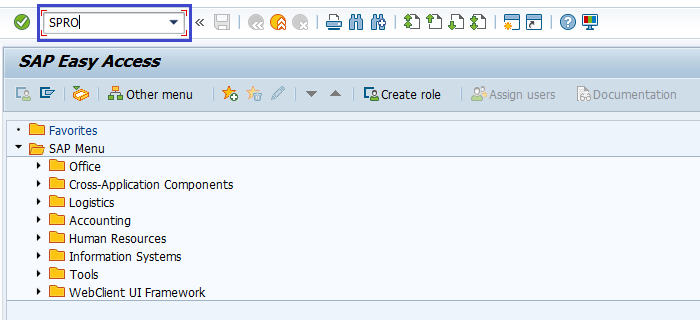Click on SAP Reference IMG.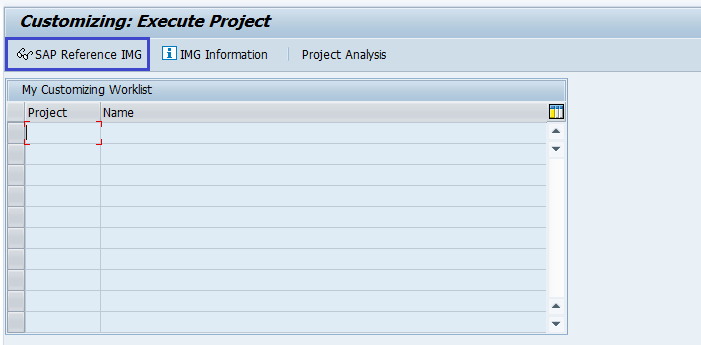Expand SAP Customizing Implementation Guide → Financial Accounting (New) → Accounts Receivable and Accounts Payable → Business Transactions → Interest Calculation → Interest Calculation Global Settings → Define Interest Calculation Types . Click on Execute.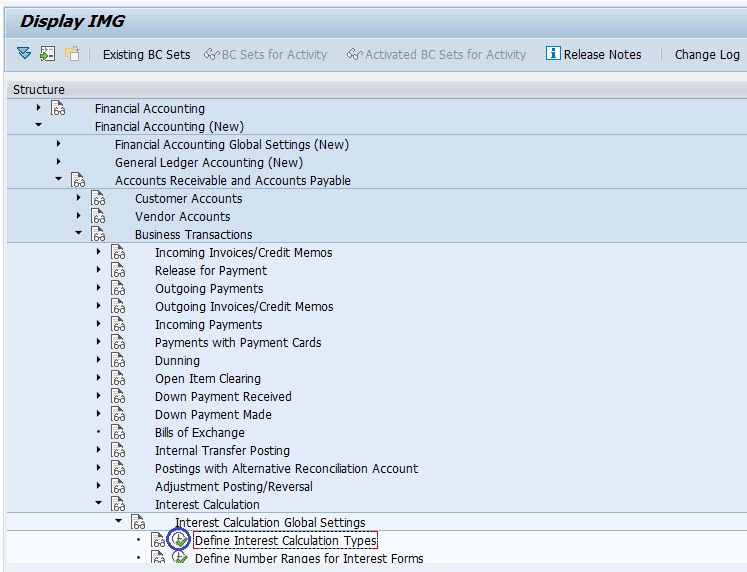Step-2: In the next screen, select activity New Entries on the application bar.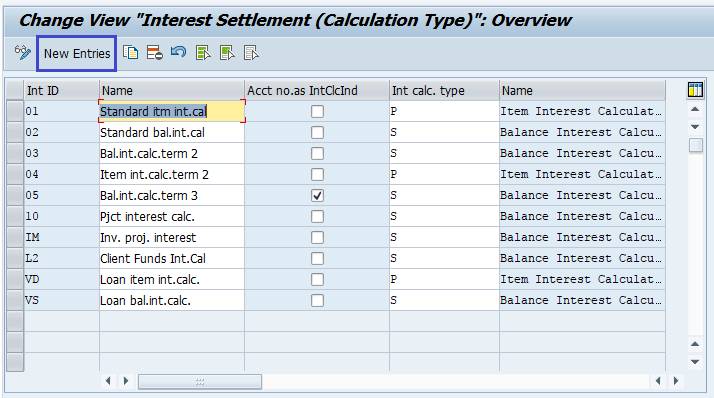Step-3: In the next screen, enter the below details.

• Int ID – Specify unique interest name (2-digit alphanumeric).
• Name – Specify the description of interest type.
• Account no.as IntClcInd – Check if account number shown as interest calculation.
• Int calc. type – Specify the interest calculation type.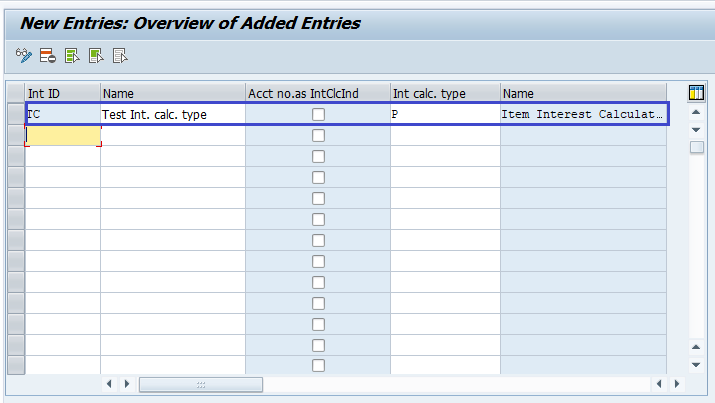Step-4: Once all the details entered, click on the Save button to save the changes.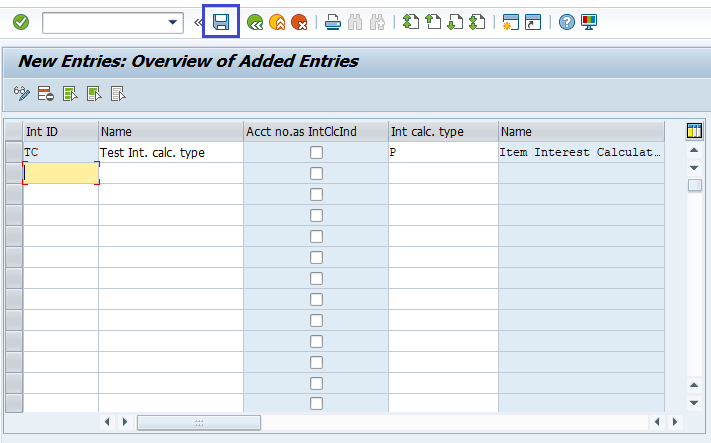Step-5: It prompt for the Change Request. Create/Select the change request and Click on right mark to proceed.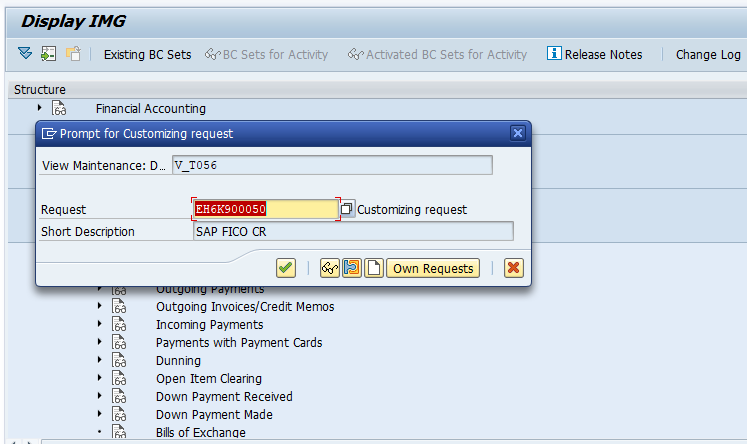Step-6: Status bar displays the below message once the interest calculation type defined successfully.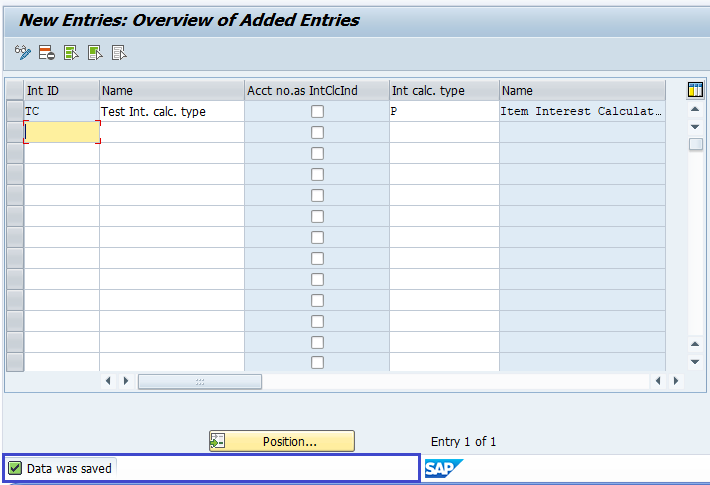### Technical Information: -

• Transaction Code: - OB46
• Navigation path: - SPRO → SAP Reference IMG → SAP Customizing Implementation Guide → Financial Accounting (New) → Accounts Receivable and Accounts Payable → Business Transactions → Interest Calculation → Interest Calculation Global Settings → Define Interest Calculation Types
• Database Table: - T056, T056X